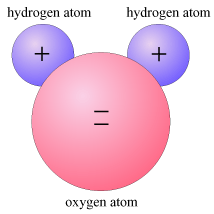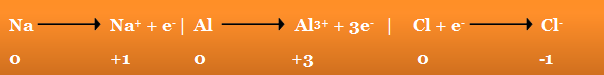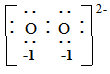×#### Thank you for registering.

One of our academic counsellors will contact you within 1 working day.

Click to Chat

1800-1023-196

+91-120-4616500

CART 0

• 0

MY CART (5)

Use Coupon: CART20 and get 20% off on all online Study Material

ITEM
DETAILS
MRP
DISCOUNT
FINAL PRICE
Total Price: Rs.

There are no items in this cart.
Continue ShoppingOxidation Number or Oxidation State

Table of Content

Common Oxidising and Reducing Agents

Oxidation State as Periodic Property

Application of Oxidation State

Oxidation State V/S Valency

Related ResourcesWhen an element is oxidised it must be acting as a reducing agent and it, therefore, loses electrons; when reduced, it gains electrons. The oxidation state or oxidation number of an element is the number of electrons it might be considered to have lost or gained.

All elements in the elementary, uncombined state are given oxidation numbers of zero. When sodium, for example, is oxidised it loses one electron, and the Na+ ion is said to have an oxidation number of +1. Similarly, the Cu2+ and Al3+ ions have oxidation numbers of +2 and +3, whilst F- and O2- have oxidation numbers of - 1 and - 2. For simple ions, the oxidation number is equal to the ionic charge, e.g.The oxidation number for an element in a covalent compound is  by taking the oxidation number to be equal to the charge that the element would carry, if all the bonds in the compound were regarded as ionic instead of covalent. In doing this, a shared pair or electrons between two atoms is assigned to the atom with the greater electronegativity. Or, if the two atoms are alike, the shared pair is split between the two, one electron being assigned to each atom. The resulting charges on the various atoms when the bonding electrons are so assigned are the oxidation numbers of the atoms.

The sum of the oxidation numbers of all the atoms in an uncharged compound is zero. In case of an ion,  the algebraic sum of the oxidation numbers of all the atoms is equal to the charge on the ion,

NH4+

OH-

SO42-

AlF63-

N

H

O

H

S

O

Al

Fe

-3

+1

-2

+1

+6

-2

+3

-1

Oxidation number of any element in its elementary state is zero.

Fluorine is the most electronegative element. Its oxidation number is always  -1 .

Oxygen after fluorine is the second most electronegative element. It  shows an oxidation state of -2 in almost all the compounds excepts seroxides and superoxides,

MgO

Fe2O3

C O2

Mn2O7

CrO3

Mg

O

Fe

O

C

O

Mn

O

Cr
O

+2

-2

+3

-2

+4

-2

+7

-2

+6
-2

In peroxides (O2–), oxygen has oxidation number –1; in superoxides (O22–), oxygen has oxidation number –1/2; and in OF2, the oxygen has an oxidation number +2.

F2 O

F

O

-1

+2the oxidation numbers in the peroxide ion being calculated by splitting the shared pair equally between the two oxygen atoms

In all compounds, except ionic metallic hydrides, the oxidation number of hydrogen is +1.

HCl

H2O

NH3

LiH

CaH2

H

Cl

H

O

N

H

Li

H

Ca
H

+1

-1

+1

-2

-3

+1

+1

-1

+2
-1

In any compounds has more than two elements, the oxidation number of any one of them may have to be obtained by first assigning reasonable oxidation numbers to the other elements.

H2SO3

KMnO4

K2Cr2O7

KClO4

H

S

O

K

Mn

O

K

Cr

O

K

Cl

O

+1

+4

-2

+1

+7

-2

+1

+6

-2

+1

+7

-2

Some elements like manganese, chromium, nitrogen and chlorine show variable oxidation states.

When an element is oxidised its oxidation number gets increased while reduction on any element decreases its oxidation number. Change in oxidation number can be used to decide whether an oxidation or a reduction has taken place. In the change from chloromethane to dichloromethane, for example,
C  H3  Cl    C  H2 Cl2
-2 +1 -1     0 +1 -1
The oxidation number of carbon is increased from -2 to 0. The carbon is therefore being oxidised.

Common Oxidising and Reducing Agents

The functioning of some common oxidising and reducing agents is summarised below

Oxidising agent

Effective Change

Decrease in Oxidation Number

KMnO4 in acid solution

MnO4 -  → Mn2+

5

KMnO4 in alkaline solution

MnO4 - →  MnO2

3

K2Cr2O7 in acid solution

Cr2O72- →  Cr3+

3

dilute HNO3

NO3- →  NO

3

concentrated HNO3

NO3- →  NO2

1

concentrated H2SO4

SO42- →   SO2

2

manganese (IV) oxide

MnO2 → Mn2+

2

chlorine

Cl →  Cl-

1

chloric (I) acid

ClO-  →  Cl-

2

KlO3 in dilute acid

IO3-  →  I

5

KlO3 in concentrated acid

IO3-   →  I-

4

Reducing agent

Effective Change

Increase in Oxidation Number

iron (II) salts (acid)

Fe2+ →  Fe3+

1

tin (II) salts (acid)

Sn2+ →  Sn4+

2

ethanedioates (acid)

C2O42- →  CO2

1

sulphites (acid)

SO32-  →SO42-

2

hydrogen sulphide

S2- → S

2

iodides (dilute acid)

I- → I

1

iodides (concentrated acid)

I- → I+

2

metals, e.g. Zn

Zn → Zn2+

2

hydrogen

H  → H+

1

Example

Question:

What is the oxidation number of Mn is KMnO4 ?

Solution:

Let the oxidation number  of Mn in KMnO4 be x.

We know that

Oxidation number of K = +1

Oxidation number = –2

(Oxidation number K) + (Oxidation number of  Mn) +4(Oxidation number of O) = 0

(+1)+(x)+4(-2) = 0

or x = +7

Oxidation State as Periodic PropertyOxidation state of an atom depends upon the electronic configuration of atom, a periodic property.

IA group or alkali metals shows +1 oxidation state.

II A group or alkali earth metals show +2 oxidation state

The maximum normal oxidation state, shown by III A group elements is +3. These elements also show +2 to +1 oxidation states.

Elements of IV A group show their max. and min. oxidation states +4 and -4 respectively.

Non-metals show number of oxidation states, the relation between max. and mini. oxidation states for non metals is equal to maximum oxidation state - minimum oxidation state =8
For example S has maximum oxidation number +6 as being VI Agroup element.

Maximum oxidation number of an element is equal to group number – 8

I Group elements always show +1 oxidation number.

II Group elements always show +2 oxidation number.

III Group elements always show +3 oxidation number.

IV Group elements show -4 to +4 oxidation number.

V Group elements show -3 to +5 oxidation number.

VI Group elements show -2 to +6 oxidation number.

VII Group elements show -1 to +7 oxidation number.

Inert gases show zero oxidation number.

Application of Oxidation State

To compare the strength of acid and base

Strength of acids increases with increase in oxidation number.

Strength of base decreases with increase in oxidation number.

To determine the oxidising and reducing nature

Group

Range of oxidation number
(n-8) to n
[n = number of group]

IA

+1

IIA

+2

IIIA

+1,+3

IVA

-4 to +4

VA

-3 to +5

VIA

-2 to +6 [Exception → Maximum oxidation number of O is 2]

VIIA

-1 to +7 [Exception → Oxidation number of F is -1]

If any compound is in maximum oxidation state , then it will act as oxidant only.

If any compound is in minimum oxidation state, then it will act as reductant only.

If the oxidation state is intermediate, then compound can act as both reductant as well as oxidant.

To determine molecular formula of any compound

Suppose that there are three atoms A, B, C and their oxidation number are 6, -1, -2 respectively. Then the molecular formula of compound formed by them will be AB4C  because

+6 = (-14)+(-2)

or +6 = -6

Watch this Video for more reference

Oxidation State V/S Valency

Oxidation State

Valency

It represents the number of electrons which an atom of an element appears to have gained or lost when in the combined state.

It is the number of hydrogen or chlorine atoms or double the number of oxygen atoms that combine with one atom of the element

Oxidation number of an element may be different in different compounds.

Valency of an element usually remains fixed.

Oxidation state or oxidation number of an element may be positive, negative or zero.

Valency of an element is either positive or negative.

Oxidation state may have fractional values

Valenvy is always a whole number.Summary

The oxidation number (Ox.no.) of an atom in free elements is zero, no matter how complicated the molecule is, hydrogen in H2, sulphur in S8, phosphorus in P4, oxygen in O2 or O3, all have zero value of oxidation numbers.

The fluorine, which is the most electronegative element, has oxidation number –1 in all of its compounds.

Oxidation number of oxygen is –2 in all compounds except in peroxides, superoxides and oxygen fluorides. In peroxides (O2–), oxygen has oxidation number –1; in superoxides (O22–), oxygen has oxidation number –1/2; and in OF2, the oxygen has an oxidation number +2.

The oxygen number of hydrogen is +1 in all of its compounds except in metallic hydrides like NaH, BaH2, etc. Hydrogen is in –1 oxidation state in these hydrides.

The oxidation number of an ion is equal to the electrical charge present on it.

The oxidation number of IA elements (Li, Na, K, Rb, Cs and Fe) is +1 and the oxidation number IIA elements (Be, Mg, Ca, Sr, Ba and Ra) is +2.

Sum of oxidation number of all the atoms of a complex ion is equal to the net charge on the ion.

Sum of the oxidation number of all the atoms present in a neutral molecule is zero.

If any compound is in maximum oxidation state , then it will act as oxidant only.

If any compound is in minimum oxidation state, then it will act as reductant only.

If the oxidation state is intermediate, then compound can act as both reductant as well as oxidant.

I Group elements always show +1 oxidation number.

II Group elements always show +2 oxidation number.

III Group elements always show +3 oxidation number.

IV Group elements show -4 to +4 oxidation number.

V Group elements show -3 to +5 oxidation number.

VI Group elements show -2 to +6 oxidation number.

VII Group elements show -1 to +7 oxidation number.

Inert gases show zero oxidation number.Question 1: Oxidation state of Mg in MgO is

a. -1
b. -2
c. +2
d. +1

Question 2: Which of the following oxidation states are not possible for O?

a. -1

b. -2

c. -1/2

d. +3/2

Question 3: Oxidation state of O in OF2 is..

a. +2

b. -2

c. -1

d. +1

Question 4: In the case of neutral molecules, the algebraic sum of the oxidation number of all the atoms present in the molecule is.

a. zero

b. one

c. two

d. threeQ.1

Q.2

Q.3

Q.4

c

d

a

a

Related Resources

Have a look at past year papers of IIT JEE

You can refer to oxidation and reduction

To read more, Buy study materials of Redox Reactions comprising study notes, revision notes, video lectures, previous year solved questions etc. Also browse for more study materials on Chemistry here.
`### Course Features

• 731 Video Lectures
• Revision Notes
• Previous Year Papers
• Mind Map
• Study Planner
• NCERT Solutions
• Discussion Forum
• Test paper with Video Solution